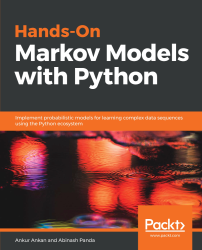•#### Hands-On Markov Models with Python#### Overview of this book

Hidden Markov Model (HMM) is a statistical model based on the Markov chain concept. Hands-On Markov Models with Python helps you get to grips with HMMs and different inference algorithms by working on real-world problems. The hands-on examples explored in the book help you simplify the process flow in machine learning by using Markov model concepts, thereby making it accessible to everyone. Once you’ve covered the basic concepts of Markov chains, you’ll get insights into Markov processes, models, and types with the help of practical examples. After grasping these fundamentals, you’ll move on to learning about the different algorithms used in inferences and applying them in state and parameter inference. In addition to this, you’ll explore the Bayesian approach of inference and learn how to apply it in HMMs. In further chapters, you’ll discover how to use HMMs in time series analysis and natural language processing (NLP) using Python. You’ll also learn to apply HMM to image processing using 2D-HMM to segment images. Finally, you’ll understand how to apply HMM for reinforcement learning (RL) with the help of Q-Learning, and use this technique for single-stock and multi-stock algorithmic trading. By the end of this book, you will have grasped how to build your own Markov and hidden Markov models on complex datasets in order to apply them to projects.
PrefaceFree Chapter
Introduction to the Markov ProcessHidden Markov ModelsState Inference - Predicting the StatesParameter Learning Using Maximum LikelihoodParameter Inference Using the Bayesian ApproachTime Series PredictingNatural Language Processing2D HMM for Image ProcessingMarkov Decision ProcessOther Books You May Enjoy# Bayesian learning in HMM

As we saw in the previous section, in the case of Bayesian learning we assume all the variables as a random variable, assign a prior to it, and then try to compute the posterior based on that. Therefore, in the case of HMM, we can assign a prior on our transition probabilities, emission probabilities, or the number of observation states.

Therefore, the first problem that we need to solve is to select the prior. Theoretically, a prior can be any distribution over the parameters of the model, but in practice, we usually try to use a conjugate prior to the likelihood, so that we have a closed-form solution to the equation. For example, in the case when the output of the HMM is discrete, a common choice of prior is the Dirichlet distribution. It is mainly for two reasons, the first of which is that the Dirichlet distribution is a conjugate distribution to...